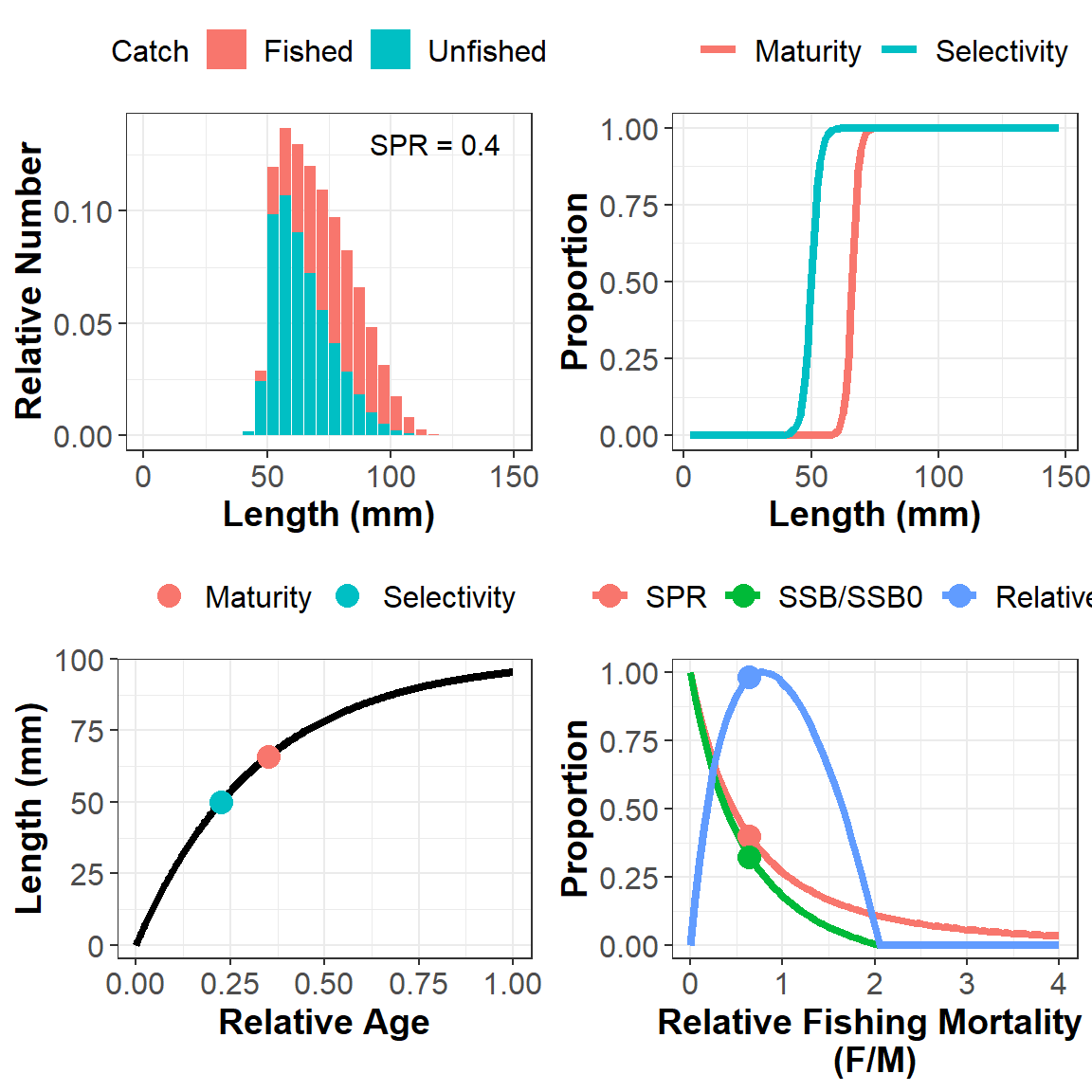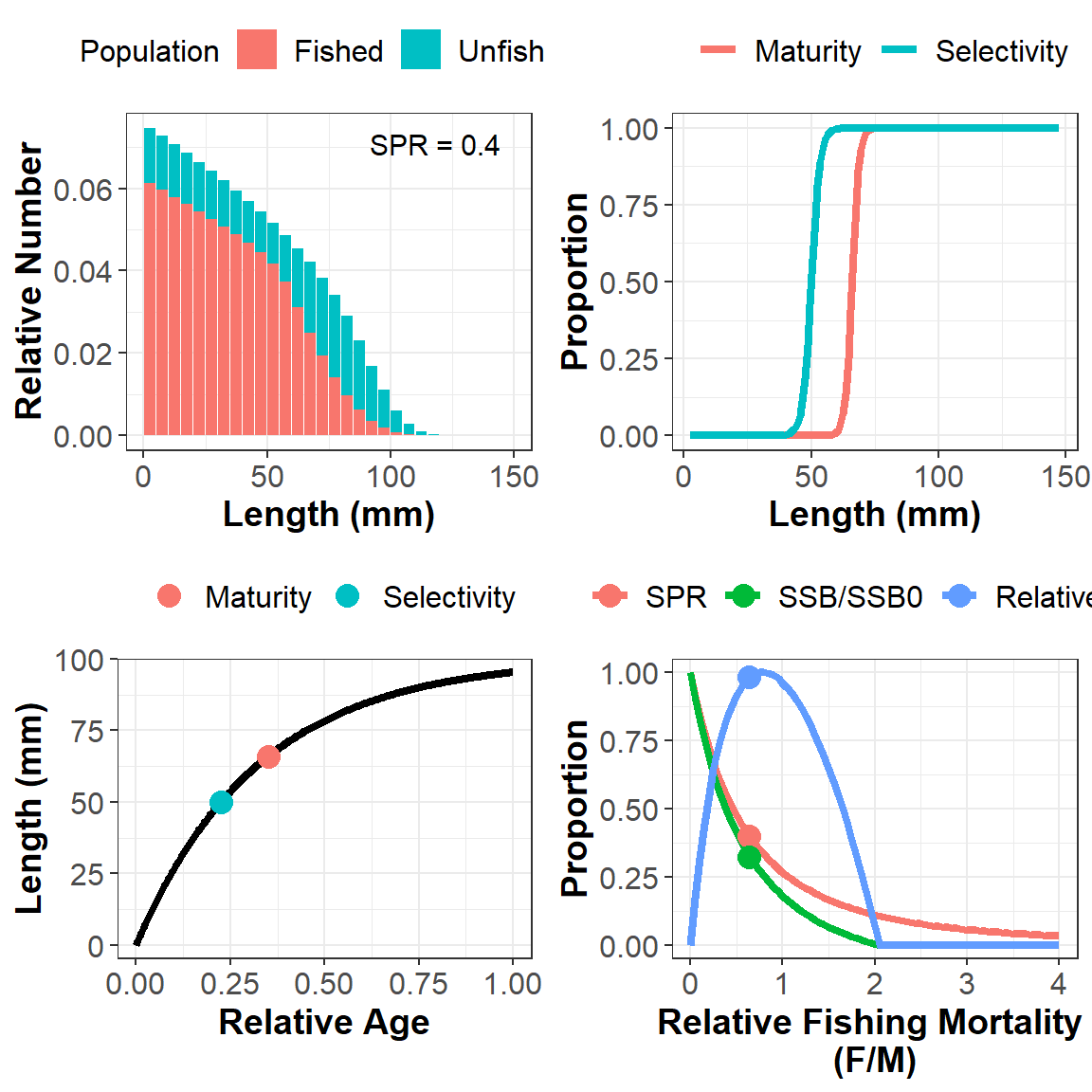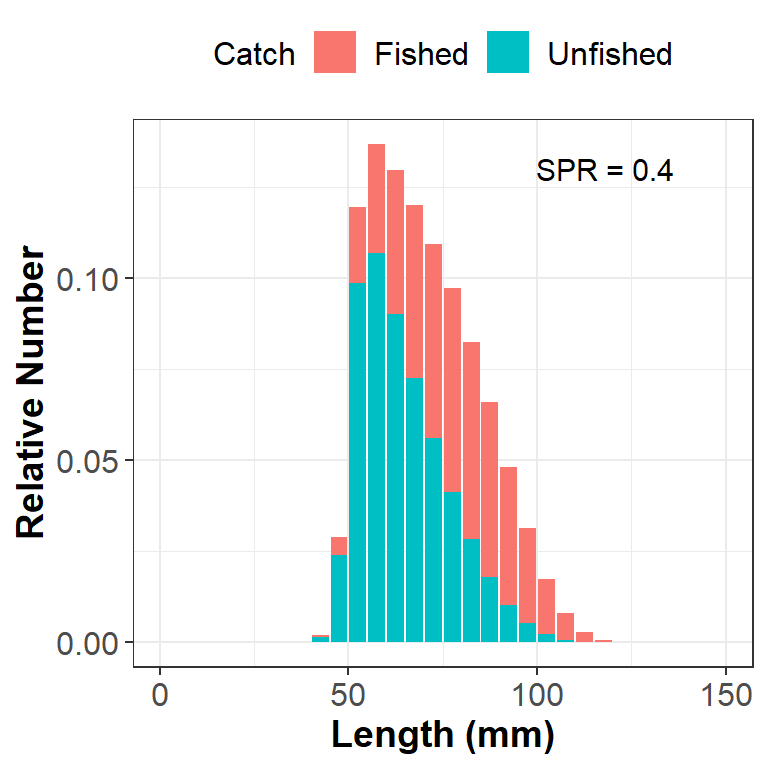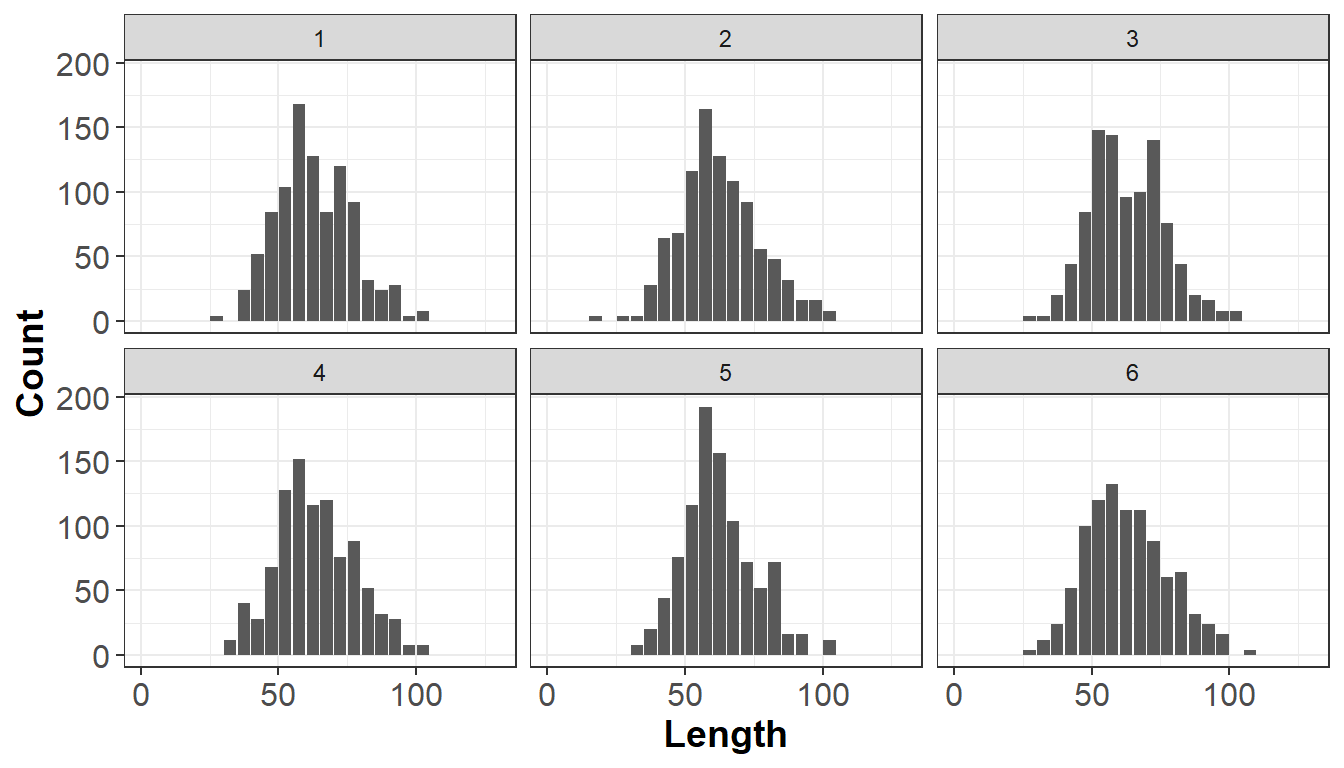# 1 Introduction

This package contains functions to run the Length-Based Spawning Potential Ratio (LBSPR) method. The LBSPR package can be used in two ways: 1) simulating the expected length composition, growth curve, and SPR and yield curves using the LBSPR model and 2) fitting to empirical length data to provide an estimate of the spawning potential ratio (SPR).

The LBSPR method has been developed for data-limited fisheries, where few data are available other than a representative sample of the size structure of the vulnerable portion of the population (i.e., the catch) and an understanding of the life history of the species. The LBSPR method does not require knowledge of the natural mortality rate (M), but instead uses the ratio of natural mortality and the von Bertalanffy growth coefficient (K) (M/K), which is believed to vary less across stocks and species than M (Prince et al. 2015).

Like any assessment method, the LBSPR model relies on a number of simplifying assumptions. In particular, the LBSPR models are equilibrium based, and assume that the length composition data is representative of the exploited population at steady state. See the publicaitons listed in the reference list for full details of the assumptions of the model, including simulation testing to evauate the effect of violations of these assumptions.

There are two versions of the LBSPR model included in this package.

## 1.1 Age-Structured Length-Based Model

The LBSPR model described by Hordyk et al. (2015a, b), and tested in a MSE framework (Hordyk et al. 2015c), use a conventional age-structured equilibrium population model. An important assumption of this model structure is that selectivity is age-based not length-based.

## 1.2 Length-Structured Growth-Type-Group Model

Hordyk et al. (2016) describe a length-structured version of the LBSPR model that uses growth-type-groups (GTG) to account for size-based selectivity. The GTG-LBSPR model also has the ability to include variable M at size (by default M is assumed to be constant). The GTG-LBSPR model typically estimates a lower fishing mortality rate for a given size structure compared to the earlier age-structured model. This is because the age-structured model has a ‘regeneration assumption’, where, because of the age-based selectivity assumption, large individuals are expected even at high fishing mortality (large, young fish).

The default setting for the LBSPR package is to use the GTG-LBSPR model for all simulation and estimation. Control options in the simulation and estimation functions can be used to switch to the age-structured LBSPR model.

## 1.3 Bug Reports

Comments and suggestions for additional features are welcome. GitHub pull requests with modifications or extensions are even more welcome!

Finally, please make sure you understand the data and the biological parameters (and how the model treats these) and critically evaluate any output of the LBSPR model.

# 2 First Steps

## 2.1 Installing the Package

The LBSPR package is now available on CRAN:

``install.packages("LBSPR")``

You can install the development version of the package from GitHub using the `devtools` package:

``````install.packages("devtools")

``library(LBSPR)``

# 3 Simulation

The LBSPR package can be used to generate the expected size composition, the SPR, and relative yield for a given set of biological and exploitation pattern parameters.

## 3.1`LB_pars` Object

The first thing to do is to create a `LB_pars` object that contains all of the required parameters for the simulation model. `LB_pars` is an S4 class object.

The S4 system is different to the S3 system that is commonly used in R, and that R users are familiar with. Don’t worry if you’ve never used S4 objects before. The main thing to know is that elements in a S4 object are called slots and you access them using the `@` symbol (rather than the `\$` symbol that is used with data.frames).

### 3.1.1 Create a new `LB_pars` Object

To create a new `LB_pars` object you use the `new` function:

``MyPars <- new("LB_pars")``
``## A blank LB_pars object created``
``## Default values have been set for some parameters``

You can see the elements or slots of the `LB_pars` object using the `slotNames` function:

``slotNames(MyPars)``
``````##   "Species"      "MK"           "M"            "Linf"
##   "L_units"      "CVLinf"       "L50"          "L95"
##   "Walpha"       "Walpha_units" "Wbeta"        "FecB"
##  "Steepness"    "Mpow"         "R0"           "SL50"
##  "SL95"         "MLL"          "sdLegal"      "fDisc"
##  "FM"           "SPR"          "BinMin"       "BinMax"
##  "BinWidth"``````

`MyPars` is an object of class `LB_pars`. You can access the help file for classes by using the `?` symbol (similar to how you find the help file for functions):

``````class?CLASSNAME

class?LB_pars``````

### 3.1.2 Populate the `LB_pars` Object

The `LB_pars` object has 25 slots. However, not all parameters need to be specified for the simulation model.

Some parameters are essential, and a warning message should appear if you attempt to progress without values (please let me know if there are issues).

Default values will be used for some of the other parameters if no value is specified. For example, the first slot (Species) is a character object that can be used for the species name. If this slot is left empty, the simulation model will populate it with a default value.

A message should alert you any time a default value is being used. Again, please let me know if you find something strange going on.

The minimum parameters that are needed for the simulation model are:

Biology

• von Bertalanffy asymptotic length (`Linf`)
• M/K ratio (natural mortality divided by von Bertalanffy K coefficient) (`MK`)
• Length at 50% maturity (`L50`)
• Length at 95% maturity (`L95`)

Exploitation - Length at 50% selectivity (`SL50`) - Length at 95% selectivity (`SL95`) - F/M ratio (`FM`) or SPR (`SPR`). If you specify both, the F/M value will be ignored.

Size Classes - Width of the length classes (`BinWidth`)

Remember, you can find the help documentation for the `LB_pars` object by typing: `class?LB_pars` in the console.

To create an example parameter object:

``````[email protected] <- 100
[email protected] <- 66
[email protected] <- 70
[email protected] <- 1.5

[email protected] <- 50
[email protected] <- 65
[email protected] <- 0.4
[email protected] <- 5``````

## 3.2 Running the Simulation Model

Now we are ready to run the LBSPR simulation model. To do this we use the `LBSPRsim` function:

``MySim <- LBSPRsim(MyPars)``
``## BinMax not set. Using default of 1.3 Linf``
``## BinMin not set. Using default value of 0``

You will notice some messages in the console alerting you that default values have been used. You can change these by specifying values in `MyPars` and re-running the `LBSPRsim` function.

We’ll manually set those values here so we don’t keep seeing the messages throughout the vignette.

``````[email protected] <- 150
[email protected] <- 0``````

We can also choose to set the units for the length parameters:

``[email protected]_units <- "mm"``

### 3.2.1 The `LB_obj` Object

The output of the `LBSPRsim` function is an object of class `LB_obj`. This is another S4 object, and contains all of the information from the `LB_pars` object and the output of the `LBSPRsim` function.

Many of the functions in the LBSPR package return an object of class `LB_obj`. You should not modify the `LB_obj` object directly. Rather, make changes to the `LB_pars` object (`MyPars` in this case), and re-run the simulation model (or other functions, covered later in the vignette).

### 3.2.2 Simulation Output

Let’s take a look at some of the simulated output.

``[email protected] ``
``##  0.4``

The simulated SPR is the same as our input value (`[email protected]`).

What is the ratio of fishing mortality to natural mortality in this scenario?

``[email protected] ``
``##  0.65``

It is important to note that the F/M ratio reported in the LBSPR model refers to the apical F over the adult natural mortality rate. That is, the value for fishing mortality refers to the highest level of F experienced by any single size class.

If the selectivity pattern excludes all but the largest individuals from being exploited, it is possible to have a very high F/M ratio in a sustainable fishery (high SPR).

A couple of more simulations with alternative values:

``````[email protected] <- numeric() # remove value for SPR
[email protected] <- 1 # set value for FM
MySim <- LBSPRsim(MyPars)
round([email protected], 2) # SPR at F/M = 1 ``````
``##  0.27``

Change the life history parameters:

``````[email protected] <- 2.0
MySim <- LBSPRsim(MyPars)
round([email protected], 2) # SPR ``````
``##  0.24``
``````[email protected] <- 0.5
MySim <- LBSPRsim(MyPars)
round([email protected], 2) # SPR ``````
``##  0.36``
``````[email protected] <- 120
MySim <- LBSPRsim(MyPars)
round([email protected], 2) # SPR ``````
``##  0.35``

Change selectivity parameters:

``````[email protected] <- 1.5
[email protected] <- 10
[email protected] <- 15
[email protected] <- 1
MySim <- LBSPRsim(MyPars)
round([email protected], 2) # SPR ``````
``##  0.13``
``````[email protected] <- 80
[email protected] <- 85
MySim <- LBSPRsim(MyPars)
round([email protected], 2) # SPR ``````
``##  0.57``

### 3.2.3 Control Options

There are a number of additional parameters that can be modified to control other aspects of the simulation model.

For example, by default the LBSPR model using the Growth-Type-Group model (Hordyk et at. 2016). The `Control` argument can be used to switch to the Age-Structured model (Hordyk et al. 2015a, b):

``````[email protected] <- 100
[email protected] <- 50
[email protected] <- 55
[email protected] <- numeric()
[email protected] <- 0.4
MySim <- LBSPRsim(MyPars, Control=list(modtype="absel"))
[email protected] ``````
``##  0.67``
``````MySim <- LBSPRsim(MyPars, Control=list(modtype="GTG"))
[email protected]  # lower F/M for the GTG model``````
``##  0.64``

See the help file for the `LBSPRsim` function for additional parameters for the `Control` argument.

### 3.2.4 Plotting the Simulation

The `plotSim` function can be used to plot `MySim`:

``plotSim(MySim) ``By default the function plots: a) the expected (equilibrium) size structure of the catch and the expected unfished size structure of the vulnerable population, b) the maturity and selectivity-at-length curves, c) the von Bertalanffy growth curve with relative age, and d) the SPR and relative yield curves as a function of relative fishing mortality (see note above on the F/M ratio).

The `plotSim` function can be controlled in a number of ways. For example, you can plot the expected unfished and fished size structure of the population by changing the `lf.type` argument:

``plotSim(MySim, lf.type="pop")``Individual plots can be created using the `type` argument:

``plotSim(MySim, type="len.freq")``See `?plotSim` for more options for plotting the output of the LBSPR simulation model.

# 4 Fitting Empirical Length Data

Two objects are required to fit the LBSPR model to length data: `LB_pars` which contains the life-history parameters (described above) and `LB_lengths`, which contains the length frequency data.

## 4.1 Creating a `LB_lengths` object

A `LB_lengths` object can be created in two ways. The `new` function can be used to create an empty object which can be manually populated:

``MyLengths <- new("LB_lengths")``
``## File not found. A blank LB_lengths object created``
``slotNames(MyLengths)``
``##  "LMids"   "LData"   "L_units" "Years"   "NYears"  "Elog"``

However, it is probably easier to create the `LB_lengths` object by directly reading in a CSV file.

A number of CSV files containing example data have been included in the LBSPR package. To find the location of the data files on your machine, use the `DataDir` function:

``datdir <- DataDir()``

The available example files can be printed out using `list.files`:

``list.files(datdir, pattern=".csv")``
``````##  "LFreq_MultiYr.csv"     "LFreq_MultiYrHead.csv" "LFreq_SingYr.csv"

The length data can be either raw data, that is, individual length measurements, or length frequency data, where the first column of the data set must contain the mid-points of the length bins, and the remaining columns contain the counts for each length class.

The data type (`freq` or `raw`) must be specified in the call to the `new` function.

A valid `LB_pars` object must also be supplied to the `new` function.

Following the normal convention in R, if a header row is present in the data file, the argument `header` in the call to `new` must be set to `TRUE`.

Finally, the full file path must be supplied to the `new` function in order to read in the CSV file. If the file is not found, or the `file` argument is left empty, a blank `LB_lengths` object with be created.

## 4.2 Reading in Example CSV

A valid `LB_pars` object must be first created (see sections above):

``MyPars <- new("LB_pars")``
``## A blank LB_pars object created``
``## Default values have been set for some parameters``
``````[email protected] <- "MySpecies"
[email protected] <- 100
[email protected] <- 66
[email protected] <- 70
[email protected] <- 1.5
[email protected]_units <- "mm"``````

Note that only the life history parameters need to be specified for the estimation model. The exploitation parameters will be estimated.

A length frequency data set with multiple years:

``````Len1 <- new("LB_lengths", LB_pars=MyPars, file=paste0(datdir, "/LFreq_MultiYr.csv"),
dataType="freq")``````

A length frequency data set with multiple years and a header row (identical to Len1 data, but with a header row):

``````Len2 <- new("LB_lengths", LB_pars=MyPars, file=paste0(datdir, "/LFreq_MultiYrHead.csv"),

A raw length data set with multiple years:

``````Len3 <- new("LB_lengths", LB_pars=MyPars, file=paste0(datdir, "/LRaw_MultiYr.csv"),
dataType="raw")``````
``## Length bin parameters (BinMax) must be set for raw data. Using defaults``
``## Length bin parameters (BinMin) must be set for raw data. Using defaults``
``## Length bin parameters (BinWidth) must be set for raw data. Using defaults``

Notice that for raw length measurements you must specify the parameters for the length bins (maximum, minimum, and width of length classes) in the `LB_pars` object. If these are left blank, default values are used.

## 4.3 Plotting Length Data

The `plotSize` function can be used to plot the imported length data. This is usually a good idea to do before proceeding with fitting the model, to confirm that everything has been read in correctly:

``plotSize(Len1)````plotSize(Len2)``